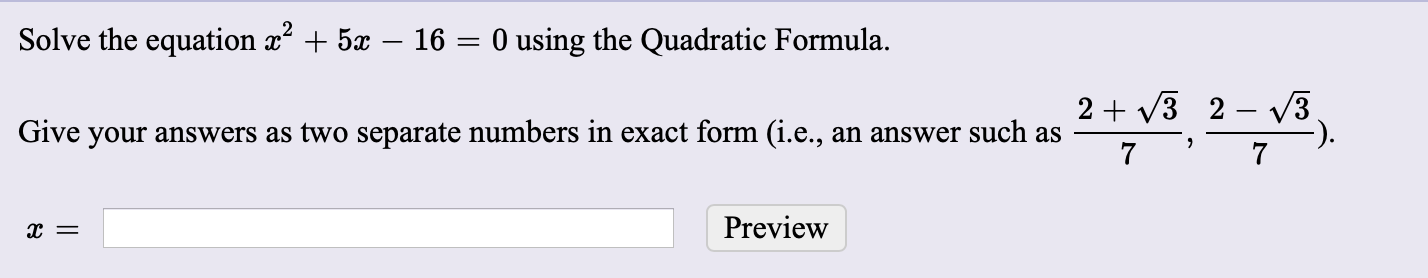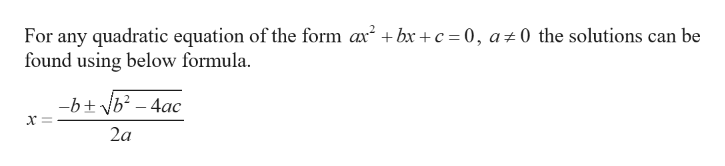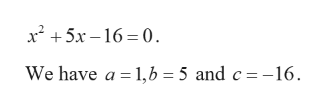# Solve the equation x + 5x – 16 = 0 using the Quadratic Formula.2 + V3 2 – 3Give your answers as two separate numbers in exact form (i.e., an answer such asPreview

Question
1 viewshelp_outlineImage TranscriptioncloseSolve the equation x + 5x – 16 = 0 using the Quadratic Formula. 2 + V3 2 – 3 Give your answers as two separate numbers in exact form (i.e., an answer such as Preview fullscreen
check_circle

Step 1help_outlineImage TranscriptioncloseFor any quadratic equation of the form ax? + bx + c = 0, a±0 the solutions can be found using below formula. -b+ vb? – 4ac 2a fullscreen
Step 2

Comparing the given equation with standard equation,help_outlineImage Transcriptionclosex² + 5x – 16 = 0. We have a = 1,6 = 5 and c=-16. fullscreen
Step 3

Substitute these values in...

### Want to see the full answer?

See Solution

#### Want to see this answer and more?

Solutions are written by subject experts who are available 24/7. Questions are typically answered within 1 hour.*

See Solution
*Response times may vary by subject and question.
Tagged in

### Equations and In-equations